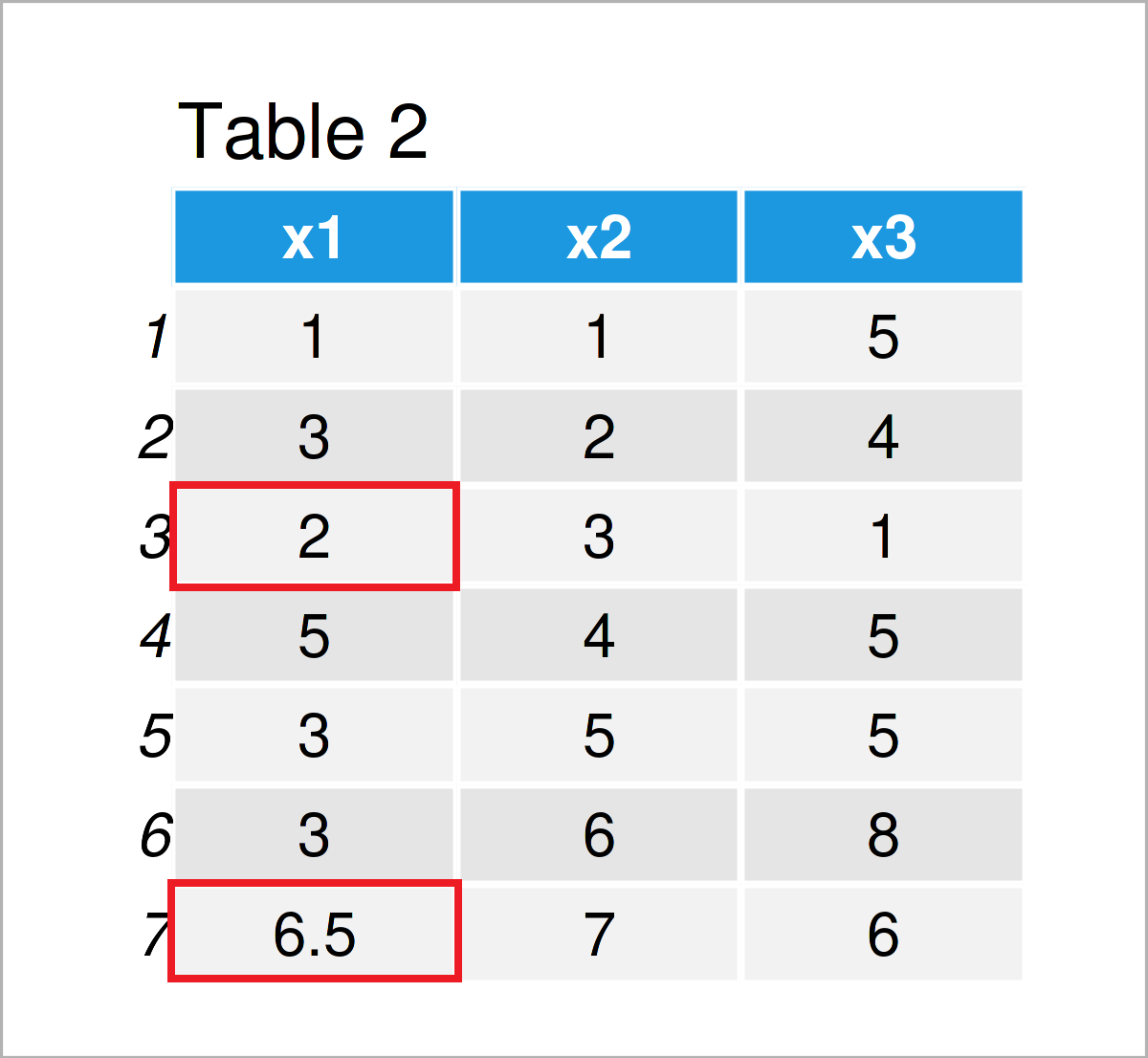# Replace NA Values by Row Mean in R (Example)

In this R programming tutorial you’ll learn how to substitute missing values by the row means.

The article looks as follows:

Here’s how to do it.

## Creation of Example Data

The first step is to create some data that we can use in the example code later on:

```data <- data.frame(x1 = c(1, 3, NA, 5, 3, 3, NA), # Create example data frame
x2 = 1:7,
x3 = c(5, 4, 1, 5, 5, 8, 6))
data                                      # Print example data frame```Table 1 shows the structure of our example data – It is constituted of seven rows and three columns. Some of the values in the column x1 are missing (i.e. NA).

The following example explains how to replace these NA values by the row means. Please be aware that such a mean imputation is usually not the best choice. Please have a look at this overview to learn more on different imputation techniques.

## Example: Substitute NA Values by Row Mean Using rowMeans() & is.na() Functions

In this example, I’ll explain how to exchange the missing values in a data frame column by the corresponding row means.

For this task, we can apply the rowMeans and is.na functions as shown below:

```data_new <- data                          # Duplicate data frame
data_new\$x1[is.na(data_new\$x1)] <- rowMeans(data_new, na.rm = TRUE)[is.na(data_new\$x1)]  # Replace by row means
data_new                                  # Print new data frame```As shown in Table 2, we have created a new data frame where the NA values have been replaced by the row means.

## Video, Further Resources & Summary

Would you like to learn more about the replacement of missing data by the corresponding row means? Then you may watch the following video on my YouTube channel. In the video, I’m explaining the R programming codes of this article in RStudio:

Please accept YouTube cookies to play this video. By accepting you will be accessing content from YouTube, a service provided by an external third party.If you accept this notice, your choice will be saved and the page will refresh.

Furthermore, you could read the related articles on my website. I have released several articles on topics such as variables, missing data, and descriptive statistics.

In summary: At this point you should have learned how to replace missing values by the corresponding row means in the R programming language.

By the way, in case you are dealing with NaN values instead of NA values, you may simply replace the is.na function by the is.nan function to substitute those values by the average. If you are dealing with NULL values, you may use the is.null function.